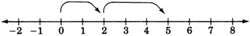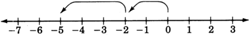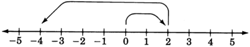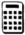# 3.3 Addition of signed numbers

 Page 1 / 1
This module is from Elementary Algebra by Denny Burzynski and Wade Ellis, Jr. The basic operations with real numbers are presented in this chapter. The concept of absolute value is discussed both geometrically and symbolically. The geometric presentation offers a visual understanding of the meaning of |x|. The symbolic presentation includes a literal explanation of how to use the definition. Negative exponents are developed, using reciprocals and the rules of exponents the student has already learned. Scientific notation is also included, using unique and real-life examples.Objectives of this module: be able to add numbers with like signs and unlike signs, understand addition with zero.

## Overview

• Addition of Numbers with Like Signs
• Addition of Numbers with Unlike Signs

## Addition of numbers with like signs

Let us add the two positive numbers 2 and 3. We perform this addition on the number line as follows.

We begin at 0, the origin.
Since 2 is positive, we move 2 units to the right.
Since 3 is positive, we move 3 more units to the right.
We are now located at 5.
Thus, $2+3=5$ .Summarizing, we have

$\left(2\text{\hspace{0.17em}}\text{positive}\text{\hspace{0.17em}}\text{units}\right)+\left(3\text{\hspace{0.17em}}\text{positive}\text{\hspace{0.17em}}\text{units}\right)=\left(5\text{\hspace{0.17em}}\text{positive}\text{\hspace{0.17em}}\text{units}\right)$

Now let us add the two negative numbers $-2$ and $-3$ . We perform this addition on the number line as follows.

We begin at 0, the origin.
Since $-2$ is negative, we move 2 units to the left.
Since $-3$ is negative, we move 3 more units to the left.
We are now located at $-5$ .

Thus, $\left(-2\right)+\left(-3\right)=-5$ .Summarizing, we have

$\left(2\text{\hspace{0.17em}}\text{negative}\text{\hspace{0.17em}}\text{units}\right)+\left(3\text{\hspace{0.17em}}\text{negative}\text{\hspace{0.17em}}\text{units}\right)=\left(5\text{\hspace{0.17em}}\text{negative}\text{\hspace{0.17em}}\text{units}\right)$

These two examples suggest that

$\begin{array}{l}\left(\text{positive}\text{\hspace{0.17em}}\text{number}\right)\text{\hspace{0.17em}}+\text{\hspace{0.17em}}\left(\text{positive}\text{\hspace{0.17em}}\text{number}\right)\text{\hspace{0.17em}}=\text{\hspace{0.17em}}\left(\text{positive}\text{\hspace{0.17em}}\text{number}\right)\\ \left(\text{negative}\text{\hspace{0.17em}}\text{number}\right)\text{\hspace{0.17em}}+\text{\hspace{0.17em}}\left(\text{negative}\text{\hspace{0.17em}}\text{number}\right)\text{\hspace{0.17em}}=\text{\hspace{0.17em}}\left(\text{negative}\text{\hspace{0.17em}}\text{number}\right)\end{array}$

## Adding numbers with the same sign

To add two real numbers that have the same sign, add the absolute values of the numbers and associate the common sign with the sum.

## Sample set a

Find the sums.

$3+7$

$\begin{array}{l}\begin{array}{cc}& \text{\hspace{0.17em}}\text{\hspace{0.17em}}\text{\hspace{0.17em}}\text{\hspace{0.17em}}\text{\hspace{0.17em}}\text{\hspace{0.17em}}\text{\hspace{0.17em}}\text{\hspace{0.17em}}\text{\hspace{0.17em}}\text{\hspace{0.17em}}\text{\hspace{0.17em}}\text{\hspace{0.17em}}\text{\hspace{0.17em}}\text{\hspace{0.17em}}\text{\hspace{0.17em}}\text{\hspace{0.17em}}\text{\hspace{0.17em}}\text{\hspace{0.17em}}\text{\hspace{0.17em}}\text{\hspace{0.17em}}\text{\hspace{0.17em}}\text{\hspace{0.17em}}\text{\hspace{0.17em}}\text{\hspace{0.17em}}\text{\hspace{0.17em}}\text{\hspace{0.17em}}\text{\hspace{0.17em}}\text{\hspace{0.17em}}\text{\hspace{0.17em}}\text{\hspace{0.17em}}\text{\hspace{0.17em}}\text{\hspace{0.17em}}\text{Add}\text{\hspace{0.17em}}\text{these}\text{\hspace{0.17em}}\text{absolute}\text{\hspace{0.17em}}\text{values}\text{.}\end{array}\\ \begin{array}{cc}\begin{array}{l}|3|=3\\ |7|=7\end{array}\right\}& \text{\hspace{0.17em}}\text{\hspace{0.17em}}\text{\hspace{0.17em}}\text{\hspace{0.17em}}\text{\hspace{0.17em}}\text{\hspace{0.17em}}\text{\hspace{0.17em}}3+7=10\end{array}\\ \begin{array}{cc}& \text{\hspace{0.17em}}\text{\hspace{0.17em}}\text{\hspace{0.17em}}\text{\hspace{0.17em}}\text{\hspace{0.17em}}\text{\hspace{0.17em}}\text{\hspace{0.17em}}\text{\hspace{0.17em}}\text{\hspace{0.17em}}\text{\hspace{0.17em}}\text{\hspace{0.17em}}\text{\hspace{0.17em}}\text{\hspace{0.17em}}\text{\hspace{0.17em}}\text{\hspace{0.17em}}\text{\hspace{0.17em}}\text{\hspace{0.17em}}\text{\hspace{0.17em}}\text{\hspace{0.17em}}\text{\hspace{0.17em}}\text{\hspace{0.17em}}\text{\hspace{0.17em}}\text{\hspace{0.17em}}\text{\hspace{0.17em}}\text{\hspace{0.17em}}\text{\hspace{0.17em}}\text{\hspace{0.17em}}\text{\hspace{0.17em}}\text{\hspace{0.17em}}\text{\hspace{0.17em}}\text{\hspace{0.17em}}\text{\hspace{0.17em}}\text{The}\text{\hspace{0.17em}}\text{common}\text{\hspace{0.17em}}\text{sign}\text{\hspace{0.17em}}\text{is}\text{\hspace{0.17em}}\text{"+}\text{."}\end{array}\end{array}$

$3+7=+10\text{\hspace{0.17em}}\text{\hspace{0.17em}}\text{\hspace{0.17em}}\text{\hspace{0.17em}}\text{\hspace{0.17em}}\text{\hspace{0.17em}}\text{\hspace{0.17em}}\text{\hspace{0.17em}}\text{\hspace{0.17em}}\text{\hspace{0.17em}}\text{\hspace{0.17em}}\text{or}\text{\hspace{0.17em}}\text{\hspace{0.17em}}\text{\hspace{0.17em}}\text{\hspace{0.17em}}\text{\hspace{0.17em}}\text{\hspace{0.17em}}\text{\hspace{0.17em}}\text{\hspace{0.17em}}\text{\hspace{0.17em}}\text{\hspace{0.17em}}\text{\hspace{0.17em}}3+7=10$

$\left(-4\right)+\left(-9\right)$

$\begin{array}{l}\begin{array}{cc}& \text{\hspace{0.17em}}\text{\hspace{0.17em}}\text{\hspace{0.17em}}\text{\hspace{0.17em}}\text{\hspace{0.17em}}\text{\hspace{0.17em}}\text{\hspace{0.17em}}\text{\hspace{0.17em}}\text{\hspace{0.17em}}\text{\hspace{0.17em}}\text{\hspace{0.17em}}\text{\hspace{0.17em}}\text{\hspace{0.17em}}\text{\hspace{0.17em}}\text{\hspace{0.17em}}\text{\hspace{0.17em}}\text{\hspace{0.17em}}\text{\hspace{0.17em}}\text{\hspace{0.17em}}\text{\hspace{0.17em}}\text{\hspace{0.17em}}\text{\hspace{0.17em}}\text{\hspace{0.17em}}\text{\hspace{0.17em}}\text{\hspace{0.17em}}\text{\hspace{0.17em}}\text{\hspace{0.17em}}\text{\hspace{0.17em}}\text{\hspace{0.17em}}\text{\hspace{0.17em}}\text{\hspace{0.17em}}\text{\hspace{0.17em}}\text{\hspace{0.17em}}\text{\hspace{0.17em}}\text{\hspace{0.17em}}\text{Add}\text{\hspace{0.17em}}\text{these}\text{\hspace{0.17em}}\text{absolute}\text{\hspace{0.17em}}\text{values}\text{.}\end{array}\\ \begin{array}{cc}\begin{array}{l}|-4|=4\\ |-9|=9\end{array}\right\}& \text{\hspace{0.17em}}\text{\hspace{0.17em}}\text{\hspace{0.17em}}\text{\hspace{0.17em}}\text{\hspace{0.17em}}\text{\hspace{0.17em}}\text{\hspace{0.17em}}4+9=13\end{array}\\ \begin{array}{cc}& \text{\hspace{0.17em}}\text{\hspace{0.17em}}\text{\hspace{0.17em}}\text{\hspace{0.17em}}\text{\hspace{0.17em}}\text{\hspace{0.17em}}\text{\hspace{0.17em}}\text{\hspace{0.17em}}\text{\hspace{0.17em}}\text{\hspace{0.17em}}\text{\hspace{0.17em}}\text{\hspace{0.17em}}\text{\hspace{0.17em}}\text{\hspace{0.17em}}\text{\hspace{0.17em}}\text{\hspace{0.17em}}\text{\hspace{0.17em}}\text{\hspace{0.17em}}\text{\hspace{0.17em}}\text{\hspace{0.17em}}\text{\hspace{0.17em}}\text{\hspace{0.17em}}\text{\hspace{0.17em}}\text{\hspace{0.17em}}\text{\hspace{0.17em}}\text{\hspace{0.17em}}\text{\hspace{0.17em}}\text{\hspace{0.17em}}\text{\hspace{0.17em}}\text{\hspace{0.17em}}\text{\hspace{0.17em}}\text{\hspace{0.17em}}\text{\hspace{0.17em}}\text{\hspace{0.17em}}\text{\hspace{0.17em}}\text{The}\text{\hspace{0.17em}}\text{common}\text{\hspace{0.17em}}\text{sign}\text{\hspace{0.17em}}\text{is}\text{\hspace{0.17em}}\text{"}-\text{."}\end{array}\end{array}$

$\left(-4\right)+\left(-9\right)=-13$

## Practice set a

Find the sums.

$8+6$

14

$41+11$

52

$\left(-4\right)+\left(-8\right)$

$-12$

$\left(-36\right)+\left(-9\right)$

$-45$

$-14+\left(-20\right)$

$-34$

$-\frac{2}{3}+\left(-\frac{5}{3}\right)$

$-\frac{7}{3}$

$-2.8+\left(-4.6\right)$

$-7.4$

Notice that

$\begin{array}{l}\left(\text{0}\right)\text{\hspace{0.17em}}+\text{\hspace{0.17em}}\left(\text{a}\text{\hspace{0.17em}}\text{positive}\text{\hspace{0.17em}}\text{number}\right)\text{\hspace{0.17em}}=\text{\hspace{0.17em}}\left(\text{that}\text{\hspace{0.17em}}\text{same}\text{\hspace{0.17em}}\text{​}\text{positive}\text{\hspace{0.17em}}\text{number}\right)\\ \left(\text{0}\right)\text{\hspace{0.17em}}+\text{\hspace{0.17em}}\left(\text{a}\text{\hspace{0.17em}}\text{negative}\text{\hspace{0.17em}}\text{number}\right)\text{\hspace{0.17em}}=\text{\hspace{0.17em}}\left(\text{that}\text{\hspace{0.17em}}\text{same}\text{\hspace{0.17em}}\text{​}\text{negative}\text{\hspace{0.17em}}\text{number)}\end{array}$

## The additive identity is 0

Since adding 0 to a real number leaves that number unchanged, 0 is called the additive identity .

## Addition of numbers with unlike signs

Now let us perform the addition $2+\left(-6\right)$ . These two numbers have unlike signs. This type of addition can also be illustrated using the number line.

We begin at 0, the origin.
Since 2 is positive, we move 2 units to the right.
Since $-6$ is negative, we move, from the 2, 6 units to the left.
We are now located at $-4$ .A rule for adding two numbers that have unlike signs is suggested by noting that if the signs are disregarded, 4 can be obtained from 2 and 6 by subtracting 2 from 6. But 2 and 6 are precisely the absolute values of 2 and $-6$ . Also, notice that the sign of the number with the larger absolute value is negative and that the sign of the resulting sum is negative.

## Adding numbers with unlike signs

To add two real numbers that have unlike signs, subtract the smaller absolute value from the larger absolute value and associate the sign of the number with the larger absolute value with this difference.

## Sample set b

Find the following sums.

$7+\left(-2\right)$

$\begin{array}{cc}\underset{\begin{array}{l}\text{Larger}\text{\hspace{0.17em}}\text{absolute}\text{\hspace{0.17em}}\text{value}\text{.}\\ \text{Sign}\text{\hspace{0.17em}}\text{\hspace{0.17em}}\text{is}\text{\hspace{0.17em}}"+".\end{array}}{\underbrace{|7|=7}}\text{\hspace{0.17em}}\text{\hspace{0.17em}}\text{\hspace{0.17em}}& \underset{\text{Smaller}\text{\hspace{0.17em}}\text{absolute}\text{\hspace{0.17em}}\text{value}\text{.}}{\underbrace{|-2|=2}}\end{array}$

$\begin{array}{ll}\text{Subtract}\text{\hspace{0.17em}}\text{absolute}\text{\hspace{0.17em}}\text{values:}\hfill & 7-2=5.\hfill \\ \text{Attach}\text{\hspace{0.17em}}\text{the}\text{\hspace{0.17em}}\text{proper}\text{\hspace{0.17em}}\text{sign:}\hfill & "+".\hfill \end{array}$

$7+\left(-2\right)=+5\text{\hspace{0.17em}}\text{\hspace{0.17em}}\text{\hspace{0.17em}}\text{\hspace{0.17em}}\text{\hspace{0.17em}}\text{\hspace{0.17em}}\text{\hspace{0.17em}}\text{\hspace{0.17em}}\text{\hspace{0.17em}}\text{\hspace{0.17em}}\text{or}\text{\hspace{0.17em}}\text{\hspace{0.17em}}\text{\hspace{0.17em}}\text{\hspace{0.17em}}\text{\hspace{0.17em}}\text{\hspace{0.17em}}\text{\hspace{0.17em}}\text{\hspace{0.17em}}\text{\hspace{0.17em}}\text{\hspace{0.17em}}7+\left(-2\right)=5$

$3+\left(-11\right)$

$\begin{array}{cc}\underset{\text{Smaller}\text{\hspace{0.17em}}\text{absolute}\text{\hspace{0.17em}}\text{value}\text{.}}{\underbrace{|3|=3}}\text{\hspace{0.17em}}\text{\hspace{0.17em}}\text{\hspace{0.17em}}& \underset{\begin{array}{l}\text{Larger}\text{\hspace{0.17em}}\text{absolute}\text{\hspace{0.17em}}\text{value}\text{.}\\ \text{Sign}\text{\hspace{0.17em}}\text{is}\text{\hspace{0.17em}}"-".\end{array}}{\underbrace{|-11|=11}}\end{array}$

$\begin{array}{ll}\text{Subtract}\text{\hspace{0.17em}}\text{absolute}\text{\hspace{0.17em}}\text{values:}\hfill & 11-3=8.\hfill \\ \text{Attach}\text{\hspace{0.17em}}\text{the}\text{\hspace{0.17em}}\text{proper}\text{\hspace{0.17em}}\text{sign:}\hfill & "-".\hfill \end{array}$

$3+\left(-11\right)=-8$

The morning temperature on a winter's day in Lake Tahoe was $-12$ degrees. The afternoon temperature was 25 degrees warmer. What was the afternoon temperature?

We need to find $-12+25$ .

$\begin{array}{cc}\underset{\text{Smaller}\text{\hspace{0.17em}}\text{absolute}\text{\hspace{0.17em}}\text{value}\text{.}}{\underbrace{|-12|=12}}\text{\hspace{0.17em}}\text{\hspace{0.17em}}\text{\hspace{0.17em}}& \underset{\begin{array}{l}\text{Larger}\text{​}\text{\hspace{0.17em}}\text{absolute}\text{\hspace{0.17em}}\text{value}\text{.}\\ \text{Sign}\text{\hspace{0.17em}}\text{is}\text{\hspace{0.17em}}\text{"+"}\text{.}\end{array}}{\underbrace{|25|=25}}\end{array}$

$\begin{array}{ll}\text{Subtract}\text{\hspace{0.17em}}\text{absolute}\text{\hspace{0.17em}}\text{values:}\hfill & 25-12=13.\hfill \\ \text{Attach}\text{\hspace{0.17em}}\text{the}\text{\hspace{0.17em}}\text{proper}\text{\hspace{0.17em}}\text{sign:}\hfill & "+".\hfill \end{array}$

$-12+25=13$

Thus, the afternoon temperature is 13 degrees.Add $-147+84$ .                                                      Display Reads

$\begin{array}{l}\begin{array}{ccc}\text{Type}& \text{\hspace{0.17em}}\text{\hspace{0.17em}}\text{\hspace{0.17em}}\text{\hspace{0.17em}}\text{\hspace{0.17em}}147& \text{\hspace{0.17em}}\text{\hspace{0.17em}}\text{\hspace{0.17em}}\text{\hspace{0.17em}}\text{\hspace{0.17em}}\text{\hspace{0.17em}}\text{\hspace{0.17em}}\text{\hspace{0.17em}}147\end{array}\\ \begin{array}{ccc}\text{Press}& \text{\hspace{0.17em}}\text{\hspace{0.17em}}\text{\hspace{0.17em}}\begin{array}{||}\hline +/-\\ \hline\end{array}& \text{\hspace{0.17em}}\text{\hspace{0.17em}}-\text{147}\end{array}\\ \begin{array}{ccc}\text{Press}& \text{\hspace{0.17em}}\text{\hspace{0.17em}}\text{\hspace{0.17em}}\begin{array}{||}\hline +\\ \hline\end{array}& \text{\hspace{0.17em}}\text{\hspace{0.17em}}\text{\hspace{0.17em}}\text{\hspace{0.17em}}\text{\hspace{0.17em}}\text{\hspace{0.17em}}\text{\hspace{0.17em}}\text{\hspace{0.17em}}\text{\hspace{0.17em}}\text{\hspace{0.17em}}-\text{147}\end{array}\\ \begin{array}{ccc}\text{Type}& \text{\hspace{0.17em}}\text{\hspace{0.17em}}\text{\hspace{0.17em}}\text{\hspace{0.17em}}\text{\hspace{0.17em}}\text{\hspace{0.17em}}\text{\hspace{0.17em}}84& \text{\hspace{0.17em}}\text{\hspace{0.17em}}\text{\hspace{0.17em}}\text{\hspace{0.17em}}\text{\hspace{0.17em}}\text{\hspace{0.17em}}\text{\hspace{0.17em}}\text{\hspace{0.17em}}\text{\hspace{0.17em}}84\end{array}\\ \begin{array}{ccc}\text{Press}& \text{\hspace{0.17em}}\text{\hspace{0.17em}}\text{\hspace{0.17em}}\begin{array}{||}\hline =\\ \hline\end{array}& \text{\hspace{0.17em}}\text{\hspace{0.17em}}\text{\hspace{0.17em}}\text{\hspace{0.17em}}\text{\hspace{0.17em}}\text{\hspace{0.17em}}\text{\hspace{0.17em}}\text{\hspace{0.17em}}\text{\hspace{0.17em}}\text{\hspace{0.17em}}-63\end{array}\end{array}$

## Practice set b

Find the sums.

$4+\left(-3\right)$

1

$-3+5$

2

$15+\left(-18\right)$

$-3$

$0+\left(-6\right)$

$-6$

$-26+12$

$-14$

$35+\left(-78\right)$

$-43$

$15+\left(-10\right)$

5

$1.5+\left(-2\right)$

$-0.5$

$-8+0$

$-8$

$0+\left(0.57\right)$

$0.57$

$-879+454$

$-425$

$-1345.6+\left(-6648.1\right)$

$-7993.7$

## Exercises

Find the sums for the the following problems.

$4+12$

16

$8+6$

$6+2$

8

$7+9$

$\left(-3\right)+\left(-12\right)$

$-15$

$\left(-6\right)+\left(-20\right)$

$\left(-4\right)+\left(-8\right)$

$-12$

$\left(-11\right)+\left(-8\right)$

$\left(-16\right)+\left(-8\right)$

$-24$

$\left(-2\right)+\left(-15\right)$

$14+\left(-3\right)$

11

$21+\left(-4\right)$

$14+\left(-6\right)$

8

$18+\left(-2\right)$

$10+\left(-8\right)$

2

$40+\left(-31\right)$

$\left(-3\right)+\left(-12\right)$

$-15$

$\left(-6\right)+\left(-20\right)$

$10+\left(-2\right)$

8

$8+\left(-15\right)$

$-2+\left(-6\right)$

$-8$

$-11+\left(-14\right)$

$-9+\left(-6\right)$

$-15$

$-1+\left(-1\right)$

$-16+\left(-9\right)$

$-25$

$-22+\left(-1\right)$

$0+\left(-12\right)$

$-12$

$0+\left(-4\right)$

$0+\left(24\right)$

24

$-6+1+\left(-7\right)$

$-5+\left(-12\right)+\left(-4\right)$

$-21$

$-5+5$

$-7+7$

0

$-14+14$

$4+\left(-4\right)$

0

$9+\left(-9\right)$

$84+\left(-61\right)$

23

$13+\left(-56\right)$

$452+\left(-124\right)$

328

$636+\left(-989\right)$

$1811+\left(-935\right)$

876

$-373+\left(-14\right)$

$-1221+\left(-44\right)$

$-1265$

$-47.03+\left(-22.71\right)$

$-1.998+\left(-4.086\right)$

$-6.084$

$\left[\left(-3\right)+\left(-4\right)\right]+\left[\left(-6\right)+\left(-1\right)\right]$

$\left[\left(-2\right)+\left(-8\right)\right]+\left[\left(-3\right)+\left(-7\right)\right]$

$-20$

$\left[\left(-3\right)+\left(-8\right)\right]+\left[\left(-6\right)+\left(-12\right)\right]$

$\left[\left(-8\right)+\left(-6\right)\right]+\left[\left(-2\right)+\left(-1\right)\right]$

$-17$

$\left[4+\left(-12\right)\right]+\left[12+\left(-3\right)\right]$

$\left[5+\left(-16\right)\right]+\left[4+\left(-11\right)\right]$

$-18$

$\left[2+\left(-4\right)\right]+\left[17+\left(-19\right)\right]$

$\left[10+\left(-6\right)\right]+\left[12+\left(-2\right)\right]$

14

$9+\left[\left(-4\right)+7\right]$

$14+\left[\left(-3\right)+5\right]$

16

$\left[2+\left(-7\right)\right]+\left(-11\right)$

$\left[14+\left(-8\right)\right]+\left(-2\right)$

4

In order for a small business to break even on a project, it must have sales of $21,000$ . If the amount of sales was $15,000$ , how much money did this company fall short?

Suppose a person has $56.00$ in his checking account. He deposits $100.00$ into his checking account by using the automatic teller machine. He then writes a check for $84.50$ . If an error causes the deposit not to be listed into this person's account, what is this person's checking balance?

$-28.50$

A person borrows $7.00$ on Monday and then $12.00$ on Tuesday. How much has this person borrowed?

A person borrows $11.00$ on Monday and then pays back $8.00$ on Tuesday. How much does this person owe?

$3.00$

## Exercises for review

( [link] ) Simplify $\frac{4\left({7}^{2}-6\cdot {2}^{3}\right)}{{2}^{2}}$ .

( [link] ) Simplify $\frac{35{a}^{6}{b}^{2}{c}^{5}}{7{b}^{2}{c}^{4}}$ .

$5{a}^{6}c$

( [link] ) Simplify ${\left(\frac{12{a}^{8}{b}^{5}}{4{a}^{5}{b}^{2}}\right)}^{3}$ .

( [link] ) Determine the value of $|-8|$ .

8

( [link] ) Determine the value of $\left(|2|+{|4|}^{2}\right)+{|-5|}^{2}$ .

Application of nanotechnology in medicine
what is variations in raman spectra for nanomaterials
I only see partial conversation and what's the question here!
what about nanotechnology for water purification
please someone correct me if I'm wrong but I think one can use nanoparticles, specially silver nanoparticles for water treatment.
Damian
yes that's correct
Professor
I think
Professor
what is the stm
is there industrial application of fullrenes. What is the method to prepare fullrene on large scale.?
Rafiq
industrial application...? mmm I think on the medical side as drug carrier, but you should go deeper on your research, I may be wrong
Damian
How we are making nano material?
what is a peer
What is meant by 'nano scale'?
What is STMs full form?
LITNING
scanning tunneling microscope
Sahil
how nano science is used for hydrophobicity
Santosh
Do u think that Graphene and Fullrene fiber can be used to make Air Plane body structure the lightest and strongest. Rafiq
Rafiq
what is differents between GO and RGO?
Mahi
what is simplest way to understand the applications of nano robots used to detect the cancer affected cell of human body.? How this robot is carried to required site of body cell.? what will be the carrier material and how can be detected that correct delivery of drug is done Rafiq
Rafiq
if virus is killing to make ARTIFICIAL DNA OF GRAPHENE FOR KILLED THE VIRUS .THIS IS OUR ASSUMPTION
Anam
analytical skills graphene is prepared to kill any type viruses .
Anam
what is Nano technology ?
write examples of Nano molecule?
Bob
The nanotechnology is as new science, to scale nanometric
brayan
nanotechnology is the study, desing, synthesis, manipulation and application of materials and functional systems through control of matter at nanoscale
Damian
Is there any normative that regulates the use of silver nanoparticles?
what king of growth are you checking .?
Renato
What fields keep nano created devices from performing or assimulating ? Magnetic fields ? Are do they assimilate ?
why we need to study biomolecules, molecular biology in nanotechnology?
?
Kyle
yes I'm doing my masters in nanotechnology, we are being studying all these domains as well..
why?
what school?
Kyle
biomolecules are e building blocks of every organics and inorganic materials.
Joe
anyone know any internet site where one can find nanotechnology papers?
research.net
kanaga
sciencedirect big data base
Ernesto
Introduction about quantum dots in nanotechnology
hi
Loga
what does nano mean?
nano basically means 10^(-9). nanometer is a unit to measure length.
Bharti
how did you get the value of 2000N.What calculations are needed to arrive at it
Privacy Information Security Software Version 1.1a
Good
Please keep in mind that it's not allowed to promote any social groups (whatsapp, facebook, etc...), exchange phone numbers, email addresses or ask for personal information on QuizOver's platform.ByBy RhodesBy OpenStaxBy OpenStaxBy OpenStaxBy Madison ChristianBy OpenStaxBy OpenStaxBy Stephen VoronBy JavaChamp TeamBy OpenStax